### Electric Circuit and Electron Device–10 marks (Part B) Questions with Answers

Anna University

Electric Circuit and Electron Device

Subject Code : EC2151

Subject Name : Electric Circuit and Electron Device

Year : 1st yr

Semester : 2nd Sem

Department : CSE & ECE

Important 10 MARKS (Part B) Questions with Answers

1. DETERMINE THE EQUIVALENT RESISTANCE BETWEEN TERMINALS A & B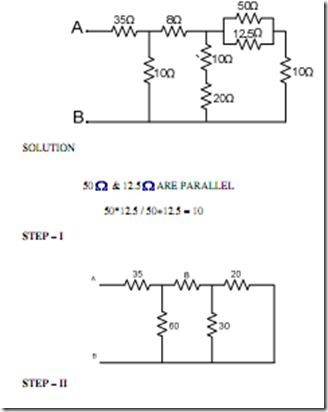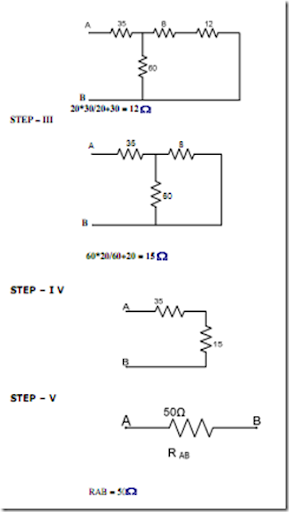2. Find the mesh currents in the following network

Solution:

The solution of -1 amp for I2 means that our initially assumed direction of current was incorrect. In actuality, I2 is flowing in a counter-clockwise direction at a value of (positive) 1 amp: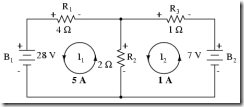3. Explain the working of a moving iron type instruments.

These instruments are widely used in laboratories and switch board at commercial frequencies because these are cheaper in cost, robust in construction and can be manufactured with required accuracy.

These are generally of two types:-

1. The attraction type.

2. The repulsion type.

The attraction type instrument operate on the principle of attraction of a single piece of soft iron into a magnetic field and repulsion type instrument operate on the principle of repulsion of two adjacent iron pieces magnified by the same magnetic field. Repulsion type instrument are more sensitive than attraction type instrument as in repulsion type instrument large separating torque is developed by having two iron element positional class together inside the field coil where the magnetizing effect is maximum.

In both type of these instruments, the current under measurement is passed through a coil of wire. This current carrying coil set up the necessary field depending on the magnitude of the current to be measured. The coil may be of a few turns of very heavy conductor or of many turns of fine wire. The instrument to be used as an ammeter is provided with a

coil of few turns of thick wire in order to have low resistance and carry large current and that to be used as a voltameter is provided with a coil of large number of turns of wire in order to have high resistance and draw as small current as possible.

4. Derive the expression for torque produced in moving iron instrument.

Let L be the self inductance corresponding to a total angular deflection of q radians and change in inductance be dL correponding to small change in deflection angel dq due to small change in current.

The change in energy of magnetic field,

dw = Td dθ

Since change in energy dE = workdone, dw

Td dθ = ½ I2dL Td = ½ I2dL/dθ

where I is in amperes, L is in Henry and θ is in Radians.

Thus toruqe is proportional to the square of the instrument current and to the rate of change of inductance with deflection.

5. An energy meter revolves 10 revolutions of disc for unit of energy. Find the number of revolutions made by it during an hour when connected across when connected 20A at 210V and 0.8 power factor leading. If energy meter revolves 350 revolutions, find the % error.

Energy consumed in one hour = VI cos φ / 1000

= 210 x 20 x 0.8 / 1000

= 3.360 kwh.

The number of revolution the meter should make it is correct = 3.360 x

registration const in revolution per kwh

= 3.360 x 100

= 336

Number of revolution actually made = 350

% error = (350-336) x 100 / 350

% error = 0.1466 %

6. Explain how following torque are produced in pmmc instrument and attracted type moving iron instruments

1. Deflecting torque

2. Control torque

3. Damping torque

1. DEFLECTING TORQUE:- The deflecting torque is produced by making use of one of the magnetic, chemical, electrostatic and electromagnetic induction effects of current or voltage and causes the moving system of the instrument to move from its zero position when the instrument is connected in an electrical circuit to measure the electrical quantity. The method of producing this torque depend upon the type of instrument. In attracting the type of instrument, this torque to equal to

Td = 1/2 I2 dL/dθ

Whereas in Pmmc instruments

Td = Bilur

Where B - magnetic density i - current flowing

l - length of coil

u - number of turn r - radius of coil

2. CONTROLLING TORQUE:- The magnitude of the movement to the moving system would be somewhat indefinite under the influence of deflecting torque unless some controlling torque exist. This torque opposes

the deflecting torque and increases with increase in deflection of the moving system without controlling system the irrespective magnitude of current and moreover, once deflected it would not return to its zero position on removing the current.

In attraction type instrument it is produced by spring control and in PMMC

too it would be produced by spring control.

3. DAMPING TORQUE:- This torque is also necessary to avoid oscillation of the moving system about it's final deflected position owing to the inertia of the moving parts and to bring the moving system to rest in it's final deflected

position quickly.

7. Briefly discuss the construction and working of a PMMC instrument.

How range is extended in moving coil ammeter and voltmeter?

CONSTRUCTION:- In a permanent magnet with soft iron pole pieces, a cylindrical iron core is mounted between the two poles of the magnet giving very narrow air gap in which the sides of a pointted light rectangular coil lies. The rectangular coil is wound of many turns of coil. The purpose of using

core is to make the field uniform and to reduce the reluctance of the magnetic circuit. A low reluctance helps to retain permeance of magnet for a longer period.

WORKING OF TORQUE EQUATION:- When the current to be measured is passed through the coil, say in the direction as shown in fig. (a), deflecting torque is produced. On account of relation between permanent magnetic field and coil magnetic field, the direction of deflecting torque can be determined by applying Fleming's left hand rule.

It is the current in amperes flowing through the coil of turns N and length l meters B is flux density in test as in air gap:-

Then, deflecting force F = BilN newtons.

If r is the distance in meters in between the centers of the coil and force F.

Then deflecting torque Td = F x r = BilNr Nm

From the above expression it is obvious that if flux density B in the air gap is constant, then

deflecting torque Td = i , Tc= Td

So, θ = i

And since q is directly proportional to current, the scale of the basic dc PMMC instrument are usually linearly spaced. Hence scale is linear.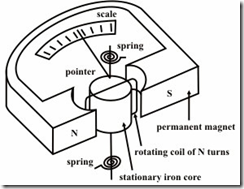8. Discuss the construction and working of an electrodynamic wattmeter with the help of diagram?

This type of instrument is similar in design and principle to the dynanometer type ammeter and voltameter.

WORKING AND CONSTRUCTION:- When the instrument of this type is used as wattmeter, the fixed coil which is divided into two equal portions in order to provide them uniform field , is employed as current coil and moving coil is used as pressure coil. The fixed coil which is divided into two equal portion in order to provide them uniform field, is employed as current coil and the moving coil is used as pressure coil, i.e the fixed coil carries the current proportional to the voltage across the circuit. A high non inductive resistance

is connected in series with the moving coil in order to limit current. The magnetic field of the fixed and moving coil react on one another causing the moving coil to turn about it's axis. The movement is controlled by hair springs which also leads the current into and out of the moving element. Damping is provided by light aluminium moving in an air dash pot. The pointer is fixed to the moving coil spindle and moves over a suitable caliberated scale.

THEORY:- Let us be the supply voltage, i the load current and R the load resistance of the moving coil circuit.

Current through fixed coil, if = i

Current through moving coil, im = V/R

deflecting torque, Td ∝ if im ∝ V/R

For a DC circuit the deflecting torque is thus proportional to the power and for any circuit with fluctuating torque. The instantaneous to the instantaneous power.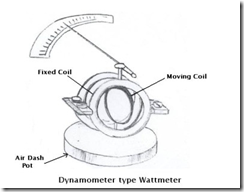9. Compare merits and demerits of moving iron type instruments and dynamometer type instruments. Which one is superior why?

1. TORQUE HEIGHT RATIO:- Dynamometer type instruments have equal small torque height ratio.

2. FRICTION ERROR:- Dynamometer type instruments have considerable friction error.

3. FRICTION LOSS:- Owing to heavy moving system, dynamometer type instruments have more friction losses.

4. COST AND SENSITIVITY TO OVERLOAD:- As a result of measures to reduce the frictional error, the dynamometer type instruments are more sensitive to overloads and mechanical impactsis in comparison to moving iron type instruments.

5. SENSTIVITY:- The senstivity of dynamometer instrument is typically very poor due to poor deflecting torque.

6. POWER CONSUMPTION:- Dynamometer type instrument have comparatively higher power consumption.

7. EFFECT OF STRAY MAGNETIC FIELD:- There is no effect of stray magnetic field on moving iron type while dynamometer type are most sensitive towards it.

8. HYSTERISIS AND EDDY CURRENT ERRORS:- Dynamometer type instruments are free from these erors while moving iron have these errors.

9. EFFECT OF WAVE FORM:- Dynamometer type instruments are very useful for accurate measurement of runs voltage while frequency change serious e rrors in AC measurement in moving iron type instruments.

10. CALIBRATION:- Dynamometer type instruments have same calibration for AC and DC measurements while moving iron type have a difference between AC and DC calibration.

10. Why shunt is usually used voltmeter and ammeter? A moving coil instrument has a resistance of 5 Ω and gives full deflection of 100mv. Show how the instrument may be used to measure:-

1. voltage upto 50V

2. current upto 10A

Shunt is usually used in voltmeter and ammeter to extend the range of voltmeter and ammeters.

Rm = 5Ω

Vm = 100mv

Im = Vm/Rm = 100mv/5Ω = 20mA

1. For measuring voltage upto 50V.

Series resistance is used with the instrument whose resistance is

R = V/Im - Rm = 50/(20 x 10-3) - 5

R = 2.5 x 10-3 - 5 = R = 2495 Ω

2. Such resistance of resistance Rf is used to be connected

Rf = Rm/[I/Im - 1]

= 5/[10/20 x 10-3 -1] = 5 x 2/998

Rf = 0.01002004 Ω

11. Explain the principle of operation of attraction type moving iron instruments and explain how the controlling and damping forces are obtained?

Answer. The earliest and simplest form of attraction moving iron instruments uses a solenoid and moving oval shaped soft iron pinoted eccentrically. To

this iron a pointer is attached so that it may deflect along with the moving iron over a graduate scale. The iron is made of sheet metal specially shaped to give a scale as nearby uniform as possible. The moving iron is drawn into field of solenoid when current flows through it. The movement of the iron always

from weaker magnetic field outside the coil into the stronger field inside the coil regardless the direction of flow of current. When the current to be measured is passed through the solenoid, a magnetic field is set up inside the solenoid, which in turn magnetises the iron. Thus the iron is attached into the coil causing the spindle and the pointer to rotate.

So much instruments normally have spring control and pneumatic damping forces.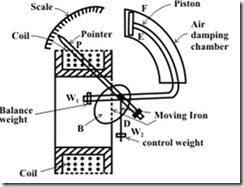12. 3-φ 4 pole induction motor is supplied from 3φ 50Hz ac supply. Find

(1) synchronous speed

(2) rotor speed when slip is 4%

(3) the rotor frequency when runs at 600r.p.m.

Sulution

1) Ns =120f/p

=120*50/4

= 1500 r.p.m.

2) speed when slip is 4% or .04

N=Ns (1-s)

=1500(1-0.04)

= 1440 r.p.m.

3) slip when motor runs at 600 r.p.m.

S’=(Ns –N)/Ns

=(1500-600)/1500

= 0.6

13. Explain the method of temperature control in open loop and closed loop systems.

Temperature controllers are needed in any situation requiring a given temperature be kept stable. This can be in a situation where an object is required to be heated, cooled or both and to remain at the target temperature (set point), regardless of the changing environment around it. There are two fundamental types of temperature control; open loop and closed loop control. Open loop is the most basic form and applies continuous heating/cooling with no regard for the actual temperature output. It is analogous to the internal heating system in a car. On a cold day, you may need to turn the heat on to full to warm the car to 75°. However, during warmer weather, the same setting would leave the inside of the car much warmer than the desired 75°.

Open loop control block diagram

Closed loop control is far more sophisticated than open loop. In a closed loop application, the output temperature is constantly measured and adjusted to maintain a constant output at the desired temperature. Closed loop control is always conscious of the output signal and will feed this back into the control process. Closed loop control is analogous to a car with internal climate control. If you set the car temperature to 75°, the climate control will automatically adjust the heating (during cold days) or cooling (during warm days) as required to maintain the target temperature of 75°.

Closed loop control block diagram

A temperature controller is a device used to hold a desired temperature at a specified value. The simplest example of a temperature controller is a common thermostat found in homes. For instance, a hot water heater uses a thermostat to control the temperature of the water and maintain it at a certain commanded temperature. Temperature controllers are also used in ovens. When a temperature is set for an oven, a controller monitors the actual temperature inside of the oven. If it falls below the set temperature, it sends a signal to activate the heater to raise the temperature back to the set point. Thermostats are also used in refrigerators. So if the temperature gets too high, a controller initiates an action to bring the temperature down.

14. Explain about open loop and closed loop control system.

Figure 1 shows an open loop system. A computed force is applied to the system which is expected to respond based on the specifications. If the system fails to respond correctly (because your estimates were off) or an unanticipated disturbance acted on it, then there is no way to correct the course. On the other hand, figure 2 shows a feed-back system. The response C(s) is measured using the sensor H(s) and the resultant is compared with the input R(s). The resultant difference (error) is acted upon by the controller which works on the actuator. The actuator then applies the required force on the system.

The closed loop thus contains the sensor dynamics, the controller dynamics, the actuator dynamics in addition to the system we are interested in. It should be noted that all measurements have to be done or converted if necessary into one unit so that comparison with the target signal is possible. Usually, measurements result in currents and voltages. Hence, this conversion from a mechanical input to an electrical output is also included in the sensor, controller and actuator dynamics.

In designing the full control system the dynamics of all the components need to be accounted for. If the controller is very slow compared to the system, it will not send the right input at the right time. In this class, we will assume perfect sensor and actuator dynamics, i.e., what goes into the sensor (it is commonly denoted by H(s)) and the actuator comes out unmodified instantaneously. So we replace them with unity transfer functions.

15. Explain the relation between voltage, current and resistance

An electric circuit is formed when a conductive path is created to allow free electrons to continuously move. This continuous movement of free electrons through the conductors of a circuit is called a current, and it is often referred to in terms of "flow," just like the flow of a liquid through a hollow pipe.

The force motivating electrons to "flow" in a circuit is called voltage. Voltage is a specific measure of potential energy that is always relative between two points. When we speak of a certain amount of voltage being present in a circuit, we are referring to the measurement of how much potential energy exists to move electrons from one particular point in that circuit to another particular point. Without reference to two particular points, the term "voltage" has no meaning.

Free electrons tend to move through conductors with some degree of friction, or opposition to motion. This opposition to motion is more properly called resistance. The amount of current in a circuit depends on the amount of voltage available to motivate the electrons, and also the amount of resistance in the circuit to oppose electron flow. Just like voltage, resistance is a quantity

relative between two points. For this reason, the quantities of voltage and resistance are often stated as being "between" or "across" two points in a circuit.

To be able to make meaningful statements about these quantities in circuits, we need to be able to describe their quantities in the same way that we might quantify mass, temperature, volume, length, or any other kind of physical quantity. For mass we might use the units of "kilogram" or "gram." For temperature we might use degrees Fahrenheit or degrees Celsius. Here are the

standard units of measurement for electrical current, voltage, and resistance:

16. Explain the construction of DC machine with neat diagram.

A D.C. machine consists mainly of two part the stationary part called stator and the rotating part called stator.

The stator consists of main poles used to produce magnetic flux ,commutating poles or interpoles in between the main poles to avoid sparking at the commutator but in the case of small machines sometimes the interpoles are avoided and finally the frame or yoke which forms the supporting structure of the machine.

The rotor consist of an armature a cylindrical metallic body or core with slots in it to place armature windings or bars, a commutator and brush gears

The magnetic flux path in a motor or generator is show below and it is called the magnetic structure of generator or motor.

Cross sectional view of a DC Machine

Frame

Frame is the stationary part of a machine on which the main poles and commutator poles are bolted and it forms the supporting structure by connecting the frame to the bed plate. The ring shaped body portion of the frame which makes the

magnetic path for the magnetic fluxes from the main poles and interpoles is called Yoke.

Yoke

In early days Yoke was made up of cast iron but now it is replaced by cast steel.

This is because cast iron is saturated by a flux density of 0.8 Wb/sq.m where as saturation with cast iron steel is about 1.5 Wb/sq.m.So for the same magnetic flux density the cross section area needed for cast steel is less than cast iron hence the weight of the machine too. If we use cast iron there may be chances of blow holes in it while casting. so now rolled steels are developed and these have consistent magnetic and mechanical properties.

End Shields or Bearings

If the armature diameter does not exceed 35 to 45 cm then in addition to poles end shields or frame head with bearing are attached to the frame. If the armature diameter is greater than 1m pedestral type bearings are mounted on the machine bed plate outside the frame. These bearings could be ball or roller type but generally plain pedestral bearings are employed. If the diameter of the armature is large a brush holder yoke is generally fixed to the frame.

17.Explain the Working of dynamometer type wattmeter

The principle of operation of the electrodynamometer-type wattmeter is the same as that for dynamo-electric machines. The deflection torque is produced by the interaction of two magnetic fluxes. One of the fluxes is produced by a fixed coil which carries a current proportional to the load current and therefore called the current coil. The other flux is created by a movable coil which carries a current proportional to the load voltage and thus called the voltage or potential coil. A high non-inductive resistance is connected to the potential coil so that its current is almost in phase with the load voltage. The control torque is provided by a control spring.

In a dynamometer type wattmeter the fixed coil (current coil) is connected in series with the load.This coil is divided in to two parts and they are kept parallel to each other. The coil is thick in cross section and has less number of turns.The moving coil (pressure coil) is connected across the load. It is thin in cross - section and has hundreds of turns. It has a non - inductive high resistance in series with it

The wattmeter is an electrodynamic instrument for measuring the electric power or the supply rate of electrical energy of any given circuit. The device consists of a pair of fixed coils, known as current coils, and a movable coil known as the potential coil. The current coils are connected in series with the circuit, while the potential coil is connected in parallel. Also, on analog wattmeters, the potential coil carries a needle that moves over a scale to indicate the measurement. A current flowing through the current coil generates an electromagnetic field around the coil. The strength of this field is proportional to the line current and in phase with it. The potential coil has, as a general rule, a high-value resistor connected in series with it to reduce the current that flows through it. The result of this arrangement is that on a dc circuit, the deflection of the needle is proportional to both the current and the voltage, thus conforming to the equation W=VA or P=EI. On an ac circuit the deflection is proportional to the average instantaneous product of voltage and current, thus measuring true power, and possibly (depending on load characteristics) showing a different reading to that obtained by simply multiplying the readings showing on a stand-alone voltmeter and a stand-alone ammeter in the same circuit. The two circuits of a wattmeter are likely to be damaged by excessive

current. The ammeter and voltmeter are both vulnerable to overheating - in case of an overload, their pointers will be driven off scale - but in the wattmeter, either or even both the current and potential circuits can overheat without the pointer approaching the end of the scale! This is because the position of the pointer depends on the power factor, voltage and current. Thus, a circuit with a low power factor will give a low reading on the wattmeter, even when both of its circuits are loaded to the maximum safety limit. Therefore, a wattmeter is rated not only in watts, but also in volts and amperes.

18. Explain the construction of transformer with neat diagram.

A transformer is an electrical device used to convert AC power at a certain voltage level to AC power at a different voltage, but at the same frequency.

The construction of a transformer includes a ferromagnetic core around which multiple coils, or windings, of wire are wrapped. The input line connects to the 'primary' coil, while the output lines connect to 'secondary' coils. The alternating current in the primary coil induces an alternating magnetic flux that 'flows' around the ferromagnetic core, changing direction during each electrical cycle. The alternating flux in the core in turn induces an alternating current in each of the secondary coils. The voltage at each of the secondary coils is directly related to the primary voltage by the turns ratio, or the number of turns in the primary coil divided by the number turns in the secondary coil. For instance, if the primary coil consists of 100 turns and carries 480 volts and a secondary coil consists of 25 turns, the secondary voltage is then: secondary voltage = (480 volts) * (25/100) = 120 volts

Two coils of wire (called windings) are wound on some type of core material. In some cases the coils of wire are wound on a cylindrical or rectangular cardboard form. In effect, the core material is air and the transformer is called an AIR-CORE TRANSFORMER. Transformers used at low frequencies, such as 50 hertz and 400 hertz, require a core of low-reluctance magnetic material, usually iron. This type of transformer is called an IRON-CORE TRANSFORMER. Most power transformers are of the iron- core type. The principle parts of a transformer and their functions are:

• The CORE, which provides a path for the magnetic lines of flux.

• The PRIMARY WINDING, which receives energy from the ac source.

• The SECONDARY WINDING, which receives energy from the primary winding and delivers it to the load.

• The ENCLOSURE, which protects the above components from dirt, moisture, and mechanical damage.

A soft-iron-core transformer is very useful where the transformer must be physically small, yet efficient. The iron-core transformer provides better power transfer than does the air-core transformer. A transformer whose core is constructed of laminated sheets of steel dissipates heat readily; thus it provides for the efficient transfer of power. The majority of transformers you will encounter in Navy equipment contain laminated- steel cores. These steel laminations are insulated with a non conducting material, such as varnish, and then formed into a core. It takes about 50 such laminations to make a core an inch thick. The purpose of the laminations is to reduce certain losses.

1.If somebody wants expert take on the main topic of blogging next I advise him/her to go to this site, continue the fussy job.
Braun Series 7 Shaver review

2.It was wondering if I could use this write-up on my other website, I will link it back to your website though.Great Thanks. Razor for Men

3.I was so excited to finally be able to purchase my instant pot for my birthday but was disappointed when it came. The first thing I noticed when I opened the lid was that it smelled like it had been used and the inner pot had water spots instant pot 6 quart

4.Pretty good post. I just stumbled upon your blog and wanted to say that I have really enjoyed reading your blog posts. Any way I'll be subscribing to your feed and I hope you post again soon. Big thanks for the useful info. fit tire electric bike

5.Pretty good post. I just stumbled upon your blog and wanted to say that I have really enjoyed reading your blog posts. Any way I'll be subscribing to your feed and I hope you post again soon. Big thanks for the useful info. my guide on RGB fans

6.Hi to everybody, here everyone is sharing such knowledge, so it’s fastidious to see this site, and I used to visit this blog daily high voltage doorknob capacitors

7.Hi to everybody, here everyone is sharing such knowledge, so it’s fastidious to see this site, and I used to visit this blog daily high voltage diode

8.I see the greatest contents on your blog and I extremely love reading them. high voltage resistor

9.Superior post, keep up with this exceptional work. It's nice to know that this topic is being also covered on this web site so cheers for taking the time to discuss this! Thanks again and again! mosfet replacement

10.Awesome article, it was exceptionally helpful! I simply began in this and I'm becoming more acquainted with it better! Cheers, keep doing awesome!
edi diodes